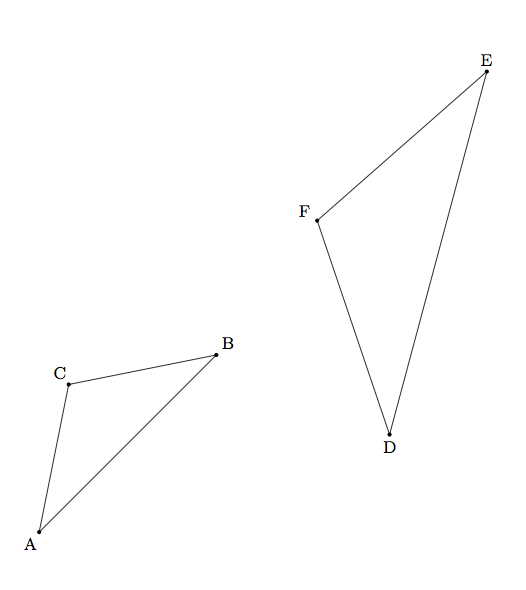# Similar triangles

Alignments to Content Standards: G-SRT.A.3

In the two triangles pictured below $m(\angle A) = m(\angle D)$ and $m(\angle B) = m(\angle E)$:Using a sequence of translations, rotations, reflections, and/or dilations, show that $\triangle ABC$ is similar to $\triangle DEF$.

## IM Commentary

This task works toward establishing the AA criterion for similarity of triangles by providing a detailed sequence of transformations that moves one of the given triangles to the other. In general, a reflection may be necessary to illustrate AA for a pair of triangles, though this is unnecessary for the given triangles (they have the same "orientation").

As with all problems where a sequence of similarity transformations is requested, there are many possibilities. Keeping to a translation followed by a rotation and dilation, as in the solution provided, we could instead translate vertex $B$ to vertex $E$ (or vertex $C$ to vertex $F$). A rotation will then align angles $ABC$ and $DEF$ (or $BCA$ and $EFD$) and then a dilation will finish showing the similarity.

The AA criterion for triangle similarity is important in the same way the ASA, SAS, and SSS criteria for triangle congruence are important. Producing an explicit series of transformations exhibiting a similarity can be difficult so when we have knowledge about the angles in triangles this is a very convenient tool.

The main mathematical practices relevant for this task are ''Reason Abstractly and Quantitatively'' (MP2) and ''Construct Viable Arguments and Critique the Reasoning of Others'' (MP3). Mathematical Practice 2 arises in producing the series of transformations exhibiting the similarity and carefully explaining the result of each operation. Mathematical Practice 3 will come up if the teacher has students compare their solutions as there are many possible series of transformations which demonstrate the similarity. If the teacher has access to graphic geometry technology, students could profitably experiment finding different ways to transform these triangles (or any other pair of similar triangles).

Writing down a careful solution can be rather technical, and notationally cumbersome, and so the task assumes a certain familiarity and confidence with similarity transformations. For students who are just beginning to experiment with these transformations, graphic geometry technology would be invaluable to build an intuition for the different steps in the construction. It would also provide students an opportunity to exhibit the similarity in different ways, for example using a sequence of reflections and then a dilation.

## Solution

We will produce a sequence of translations, rotations, reflections, and/or dilations which transform $\triangle ABC$ into $\triangle DEF$. There are many ways to do this: here we will use a translation to map $A$ to $D$, then a rotation to align two of the sides of the two triangles, and finally a dilation which completes the similarity transformation.

Step 1: Translation

The translation taking $A$ to $D$ is pictured below, with the image of $\triangle ABC$ being denoted by $A^\prime B^\prime C^\prime$.Note that $A^\prime = D$ because the translation is chosen precisely so as to map $A$ to $D$.

Step 2: Rotation

We rotate $\triangle A^\prime B^\prime C^\prime$ by angle $C^\prime A^\prime F$ as pictured below where the angle of rotation is marked in green:Also pictured is the image $A^{\prime \prime}B^{\prime \prime}C^{\prime \prime}$ of $\triangle A^\prime B^\prime C^\prime$ under this rotation. Note that $\overrightarrow{A^{\prime \prime} C^{\prime \prime}} = \overrightarrow{AC}$. This is true by the choice of our angle of rotation. Note too that $\overrightarrow{A^{\prime \prime} B^{\prime \prime}} = \overrightarrow{DE}$: this is true because $m(\angle B^{\prime \prime}A^{\prime \prime} C^{\prime \prime}) = m(\angle BAC)$, since rigid motions preserve angle measures, and $m(\angle BAC) = m(\angle EDF)$ by hypothesis.

Step 3: Dilation

We have already moved $A^{\prime \prime}$ to $D$ and so we choose $D$ as the center of our dilation. We would like to move $B^{\prime \prime}$ to $E$ and the dilation factor which will accomplish this is $\frac{|DE|}{|A^{\prime \prime} B^{\prime \prime}|}$. To check that $C^{\prime \prime}$ maps to $F$ note that $m(\angle DEF) = m(\angle A^{\prime \prime}B^{\prime \prime}C^{\prime \prime})$. Angles are preserved by dilations and so this means that $\overline{B^{\prime \prime}C^{\prime \prime}}$ must map to $\overline{EF}$ (if $C^{\prime \prime}$ mapped to a point different than $F$ then there would be two rays from $E$ to points on $\overleftrightarrow{DF}$ making the same angle with $\overrightarrow{ED}$ which is not possible).